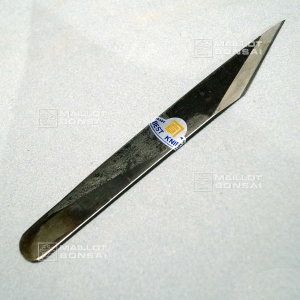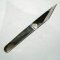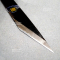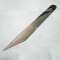##### The Japanese Bonsai specialist
Direct order Contact Help / Services Newsletter# Japanese grafting blade right handle

› Bonsai tools › Bonsai toolsref. : 10486

41,00

This item is temporarily unavailableNotify availability

###### Description

Length 180 mm, width 20 mm. Measurements of the blade: 50 mm. Made of laminated steel (layers of different kinds of steel), this traditional Japanese tool is for grafting wounds. Taking the curved handle in your hand, use your thumb to push the blade upwards against the wound. A perfectly clean cut ensures proper healing of the branch.
Don't forget to view other relevant products:

#blade 4.5 #japanese 3.8 #grafting 3.8 #handle 3.6 #bonsai 2.6 #steel 2.5 #right 2.5 #tools 2.5 #your 2.4 #measurements 2.2

Formule
(( ROUND((CHAR_LENGTH(b.article_nom)-CHAR_LENGTH(REPLACE(b.article_nom, 'blade', '')))/LENGTH('blade')) + ROUND((CHAR_LENGTH(b.article_description)-CHAR_LENGTH(REPLACE(b.article_description, 'blade', '')))/LENGTH('blade')) ) * 4.5) + (( ROUND((CHAR_LENGTH(b.article_nom)-CHAR_LENGTH(REPLACE(b.article_nom, 'japanese', '')))/LENGTH('japanese')) + ROUND((CHAR_LENGTH(b.article_description)-CHAR_LENGTH(REPLACE(b.article_description, 'japanese', '')))/LENGTH('japanese')) ) * 3.8) + (( ROUND((CHAR_LENGTH(b.article_nom)-CHAR_LENGTH(REPLACE(b.article_nom, 'grafting', '')))/LENGTH('grafting')) + ROUND((CHAR_LENGTH(b.article_description)-CHAR_LENGTH(REPLACE(b.article_description, 'grafting', '')))/LENGTH('grafting')) ) * 3.8) + (( ROUND((CHAR_LENGTH(b.article_nom)-CHAR_LENGTH(REPLACE(b.article_nom, 'handle', '')))/LENGTH('handle')) + ROUND((CHAR_LENGTH(b.article_description)-CHAR_LENGTH(REPLACE(b.article_description, 'handle', '')))/LENGTH('handle')) ) * 3.6) + (( ROUND((CHAR_LENGTH(b.article_nom)-CHAR_LENGTH(REPLACE(b.article_nom, 'right', '')))/LENGTH('right')) + ROUND((CHAR_LENGTH(b.article_description)-CHAR_LENGTH(REPLACE(b.article_description, 'right', '')))/LENGTH('right')) ) * 2.5) + (( ROUND((CHAR_LENGTH(b.article_nom)-CHAR_LENGTH(REPLACE(b.article_nom, 'steel', '')))/LENGTH('steel')) + ROUND((CHAR_LENGTH(b.article_description)-CHAR_LENGTH(REPLACE(b.article_description, 'steel', '')))/LENGTH('steel')) ) * 2.5) + (( ROUND((CHAR_LENGTH(b.article_nom)-CHAR_LENGTH(REPLACE(b.article_nom, 'your', '')))/LENGTH('your')) + ROUND((CHAR_LENGTH(b.article_description)-CHAR_LENGTH(REPLACE(b.article_description, 'your', '')))/LENGTH('your')) ) * 2.4) + (( ROUND((CHAR_LENGTH(b.article_nom)-CHAR_LENGTH(REPLACE(b.article_nom, 'measurements', '')))/LENGTH('measurements')) + ROUND((CHAR_LENGTH(b.article_description)-CHAR_LENGTH(REPLACE(b.article_description, 'measurements', '')))/LENGTH('measurements')) ) * 2.2) + (( ROUND((CHAR_LENGTH(b.article_nom)-CHAR_LENGTH(REPLACE(b.article_nom, 'traditional', '')))/LENGTH('traditional')) + ROUND((CHAR_LENGTH(b.article_description)-CHAR_LENGTH(REPLACE(b.article_description, 'traditional', '')))/LENGTH('traditional')) ) * 2.1) + (( ROUND((CHAR_LENGTH(b.article_nom)-CHAR_LENGTH(REPLACE(b.article_nom, 'different', '')))/LENGTH('different')) + ROUND((CHAR_LENGTH(b.article_description)-CHAR_LENGTH(REPLACE(b.article_description, 'different', '')))/LENGTH('different')) ) * 1.9)

## Secure payment## Delivery

Our logistic partners :